Note: The other languages of the website are Google-translated. Back to English

## 如何在Excel中计算或分配字母等级？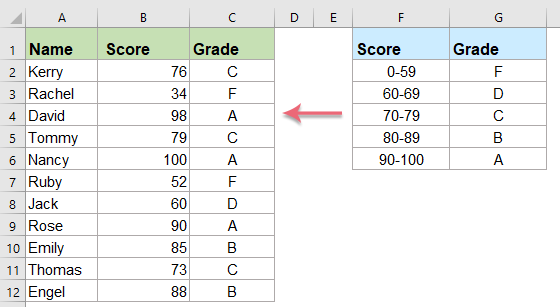#### 使用IF函数根据得分值计算字母等级

=IF (condition1, value_if_true1, IF (condition2, value_if_true2, IF (condition3, value_if_true3, value_if_false3)))
• condition1, condition2, condition3：要测试的条件。
• value_if_true1, value_if_true2, value_if_true3：如果条件的结果为TRUE，则要返回的值。
• value_if_false3：如果条件的结果为FALSE，则要返回的值。

1。 请输入以下公式或将其复制到要获取结果的空白单元格中：

=IF(B2>=90,"A",IF(B2>=80,"B",IF(B2>=70,"C",IF(B2>=60,"D","F"))))

• 如果分数（在B2单元中）等于或大于90，则学生获得A。
• 如果分数等于或大于80，则学生获得B。
• 如果分数等于或大于70，则学生获得C。
• 如果分数等于或大于60，则学生获得D。
• 否则，学生将获得F。

• B2：是您要将数字转换为字母等级的单元格。
• 数字 90, 80, 7060：是您需要分配等级的数字。

2。 然后，将填充手柄向下拖动到单元格以应用此公式，并且字母等级已显示在每个单元格中，如下所示：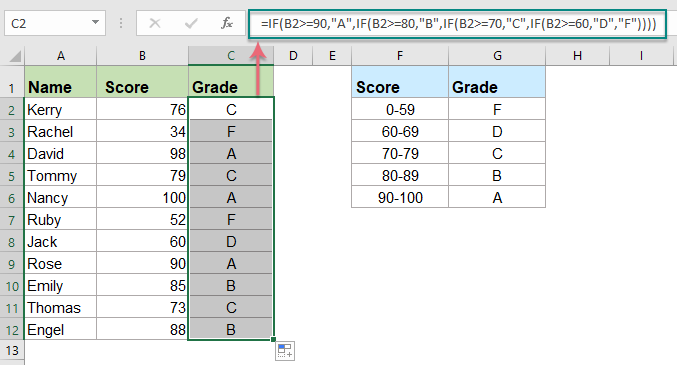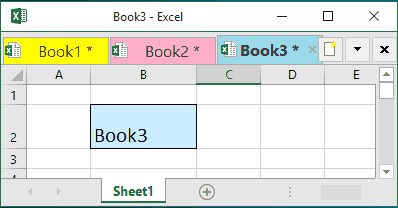## 裁员季节快到了，工作还在吗？ -- Office Tab 加快步伐，节省50％的工作时间！

•  惊人！ 多个文档的操作比单个文档更加轻松和方便。
•  与其他Web浏览器相比，Office Tab的界面更强大，更美观。
•  减少数以千计的乏味鼠标单击，告别颈椎病和鼠标手；
•  被90,000名精英和300多家知名公司选中！

#### 使用VLOOKUP函数根据得分值计算字母等级

=VLOOKUP(lookup_value, table_array, col_index_num, [range_lookup])
• lookup_value：要在table_array中搜索和找到的值。
• table_array：源表中包含您要使用的数据的单元格区域。
• col_index_num：要从中返回匹配值的table_array中的列号。
• range_lookup：值是TRUE或FALSE。
• 如果为TRUE或省略，则Vlookup返回精确或近似匹配
• 如果为假，则Vlookup只会找到完全匹配的项

1。 首先，您应该创建一个查找表，如下图所示，然后使用Vlookup函数和近似数学来获得结果。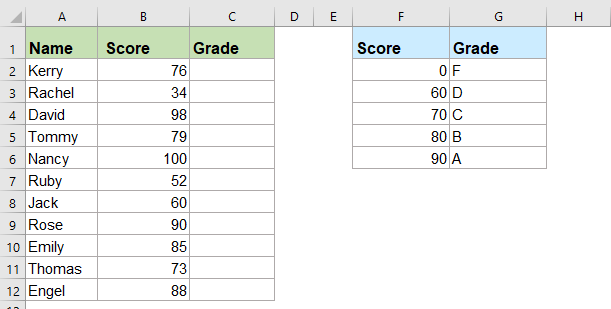2。 然后，将以下公式输入或复制到空白单元格– C3中，例如：

=VLOOKUP(B2,\$F\$2:\$G\$6,2,TRUE)

• B2：指您要计算字母等级的学生分数。
• \$ F \$ 2：\$ G \$ 6：这是返回查询值的表。
• 2：查找表中要返回匹配值的列号。
• TRUE：表示查找近似匹配值。

3。 然后，将填充手柄向下拖动到要应用此公式的单元格上，现在，您可以看到基于相应的等级比例表的所有字母等级都是一次性计算的，请参见屏幕截图：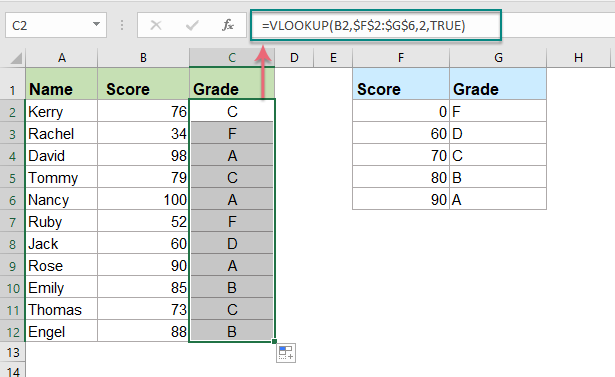#### 使用IFS功能（Excel 2019和Office 365）根据得分值计算字母等级

=IFS( logical_test1, value_if_true1, [logical_test2, value_if_true2],... )
• logical_test1：第一个条件值为TRUE或FALSE。
• value_if_true1：如果逻辑测试1为TRUE，则返回结果。 它可以是空的。
• logical_test2：计算为TRUE或FALSE的第二个条件。
• value_if_true2：如果逻辑测试2为TRUE，则返回第二个结果。 它可以是空的。

1。 请输入以下公式或将其复制到空白单元格中：

=IFS(B2>=90,"A",B2>=80,"B",B2>=70,"C",B2>=60,"D",B2<60,"F")

2。 然后，将填充手柄向下拖动到单元格以应用此公式，字母等级已显示为以下屏幕截图：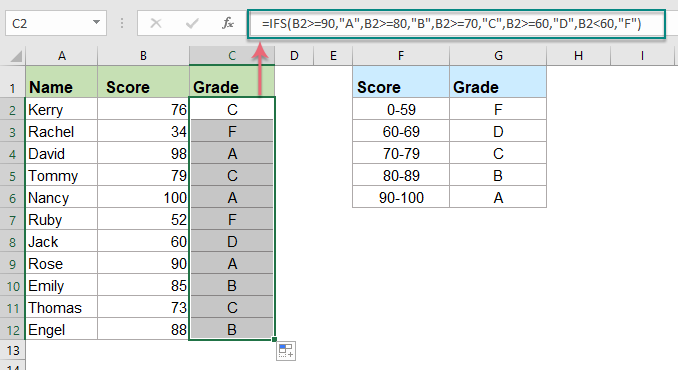#### 更多相关的文字类别文章：

• 根据Excel中的值对数据进行分类
• 假设您需要基于值对数据列表进行分类，例如，如果数据大于90，则将其分类为高；如果数据大于60且小于90，则将其分类为中；如果是，则将其分类为中。小于60，归类为“低”，您如何在Excel中解决此任务？
• 根据数字范围分配值或类别
• 本文讨论的是在Excel中分配与指定范围相关的值或类别。 例如，如果给定的数字在0到100之间，则分配值5，如果在101到500之间，则分配10，对于501到1000的范围，则分配15。本文中的方法可以帮助您实现。
• 将序列号分配给重复值或唯一值
• 如果您有一个包含一些重复项的值列表，我们是否可以为重复项或唯一值分配顺序号？ 这意味着给重复值或唯一值给出一个顺序。 本文中，我将讨论一些简单的公式来帮助您解决Excel中的这一任务。
• 在Excel中将字母等级转换为数字
• 如果您有一个包含学生姓名和字母等级的工作表，现在您想要将字母等级转换为相对数字等级，如下面的屏幕截图所示。 您可以一对一地进行转换，但是要进行大量转换却很费时间。

## 最佳办公效率工具

### Kutools for Excel解决了您的大多数问题，并使您的生产率提高了80％

• 超级公式栏 （轻松编辑多行文本和公式）； 阅读版式 （轻松读取和编辑大量单元格）； 粘贴到过滤范围...
• 合并单元格/行/列 和保存数据； 拆分单元格内容； 合并重复的行和总和/平均值...防止细胞重复； 比较范围...
• 选择重复或唯一 行; 选择空白行 （所有单元格都是空的）； 超级查找和模糊查找 在许多工作簿中； 随机选择...
• 确切的副本 多个单元格，无需更改公式参考； 自动创建参考 到多张纸； 插入项目符号，复选框等...
• 收藏并快速插入公式，范围，图表和图片； 加密单元 带密码 创建邮件列表 并发送电子邮件...
• 提取文字，添加文本，按位置删除， 删除空间; 创建和打印分页小计； 在单元格内容和注释之间转换...
• 超级滤镜 （将过滤方案保存并应用于其他工作表）； 高级排序 按月/周/日，频率及更多； 特殊过滤器 用粗体，斜体...
• 结合工作簿和工作表; 根据关键列合并表； 将数据分割成多个工作表; 批量转换xls，xlsx和PDF...
• 数据透视表分组依据 周号，周几等 显示未锁定的单元格 用不同的颜色 突出显示具有公式/名称的单元格...### Office选项卡-将选项卡式界面引入Office，使您的工作更加轻松

• 在Word，Excel，PowerPoint中启用选项卡式编辑和阅读，发布者，Access，Visio和Project。
• 在同一窗口的新选项卡中而不是在新窗口中打开并创建多个文档。
• 每天将您的工作效率提高50％，并减少数百次鼠标单击！=IF(G3<\$S\$4,\$R\$4,IF(G3<\$S\$5,\$R\$5,IF(G3<\$S\$6,\$R\$6,IF(G3<\$S\$7,\$R\$7,IF(G3<\$S\$8,\$R\$8,IF(G3<\$S\$9,\$R\$9,IF(G3<\$S\$10,\$R\$10,IF(G3<\$S\$11,\$R\$11,\$R\$12))))))))

=IF(A2>=60%," ",IF(A2>=50%,"4",IF(A2>=40%,"3",IF(A2>=30%,"2",IF(A2>=10%,"1"," ")))))

630 及更高
B 560 到 629
C 490 到 559
D 420 到 489
F 419以下

=IF(A1>=630,"A",IF(A1>=560,"B",IF(A1>=490,"C",IF(A1>=420,"D","F"))))

=IF(A2>97,"A+",IF(A2>93,"A",IF(A2>90,"A-",IF(A2>87,"B+",IF(A2>83,"B",IF(A2>80,"B-",IF(A2>77,"C+",IF(A2>73,"C",IF(A2>70,"C-",IF(A2>67,"D+",IF(A2>63,"D",IF(A2>60,"D-","F"))))))))))))

A1-91 至 100,
A2-81 至 90,
B1-71到80，
B2-61到70，
C1-51至60，
C2-41至50，
D1-35至40，
D2-0TO34。

=IF(A1>=91,"A1",IF(A1>=81,"A2",IF(A1>=71,"B1",IF(A1>=61,"B2",IF(A1>=51,"C1",IF(A1>=41,"C2",IF(A1>=35,"D1","D2")))))))

840-879 PP

880-919 议员

920-959 毫米

960-999 分

1000-1029 日

1030-1059 天*天

1060+ 天*天*

=IF(Y3>=840,AB3,IF(Y3>=880,AB4,IF(Y3>=920,AB5,IF(Y3>=960,AB6,IF(Y3>=1000,AB7,IF(Y3>=1030,AB8,IF(Y3>=1060,AB9"NYA")))))))

=IF(A1>=1060,"D*D*",IF(A1>=1030,"D*D",IF(A1>=1000,"DD",IF(A1>=960,"DM",IF(A1>=920,"MM",IF(A1>=880,"MP",IF(A1>=840,"PP","NYA")))))))

=IF(G7>=40.0,"高烧可能性",IF(G7>=37.6,"发热可能性",if(and,G7<=39.9,”发热可能性”,IF(G7>=35.1, "Ok",,if(and,G7<=37.5,”Ok” IF(B2<=35.0,"Ok","看起来很低"))))

0   产品特性

## 关注我们

Microsoft和Office徽标是Microsoft Corporation在美国和/或其他国家的商标或注册商标。